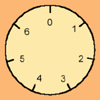# Resources tagged with: Number theory

Filter by: Content type:
Age range:
Challenge level:

### There are 23 results

Broad Topics > Properties of Numbers > Number theory### An Introduction to Modular Arithmetic

##### Age 14 to 18

An introduction to the notation and uses of modular arithmetic### More Sums of Squares

##### Age 16 to 18

Tom writes about expressing numbers as the sums of three squares.### Novemberish

##### Age 14 to 16Challenge Level

a) A four digit number (in base 10) aabb is a perfect square. Discuss ways of systematically finding this number. (b) Prove that 11^{10}-1 is divisible by 100.### Filling the Gaps

##### Age 14 to 16Challenge Level

Which numbers can we write as a sum of square numbers?### Really Mr. Bond

##### Age 14 to 16Challenge Level

115^2 = (110 x 120) + 25, that is 13225 895^2 = (890 x 900) + 25, that is 801025 Can you explain what is happening and generalise?### Modulus Arithmetic and a Solution to Differences

##### Age 16 to 18

Peter Zimmerman, a Year 13 student at Mill Hill County High School in Barnet, London wrote this account of modulus arithmetic.### The Public Key

##### Age 16 to 18Challenge Level

Find 180 to the power 59 (mod 391) to crack the code. To find the secret number with a calculator we work with small numbers like 59 and 391 but very big numbers are used in the real world for this.### An Introduction to Number Theory

##### Age 16 to 18

An introduction to some beautiful results in Number Theory.### Data Chunks

##### Age 14 to 16Challenge Level

Data is sent in chunks of two different sizes - a yellow chunk has 5 characters and a blue chunk has 9 characters. A data slot of size 31 cannot be exactly filled with a combination of yellow and. . . .### Pythagorean Golden Means

##### Age 16 to 18Challenge Level

Show that the arithmetic mean, geometric mean and harmonic mean of a and b can be the lengths of the sides of a right-angles triangle if and only if a = bx^3, where x is the Golden Ratio.### Mod 7

##### Age 16 to 18Challenge Level

Find the remainder when 3^{2001} is divided by 7.### Sums of Squares and Sums of Cubes

##### Age 16 to 18

An account of methods for finding whether or not a number can be written as the sum of two or more squares or as the sum of two or more cubes.### A Little Light Thinking

##### Age 14 to 16Challenge Level

Here is a machine with four coloured lights. Can you make two lights switch on at once? Three lights? All four lights?### There's a Limit

##### Age 14 to 18Challenge Level

Explore the continued fraction: 2+3/(2+3/(2+3/2+...)) What do you notice when successive terms are taken? What happens to the terms if the fraction goes on indefinitely?### Number Rules - OK

##### Age 14 to 16Challenge Level

Can you produce convincing arguments that a selection of statements about numbers are true?### Always Perfect

##### Age 14 to 18Challenge Level

Show that if you add 1 to the product of four consecutive numbers the answer is ALWAYS a perfect square.### Euler's Squares

##### Age 14 to 16Challenge Level

Euler found four whole numbers such that the sum of any two of the numbers is a perfect square...### Ordered Sums

##### Age 14 to 16Challenge Level

Let a(n) be the number of ways of expressing the integer n as an ordered sum of 1's and 2's. Let b(n) be the number of ways of expressing n as an ordered sum of integers greater than 1. (i) Calculate. . . .##### Age 16 to 18

What is the relationship between the arithmetic, geometric and harmonic means of two numbers, the sides of a right angled triangle and the Golden Ratio?### 2^n -n Numbers

##### Age 16 to 18

Yatir from Israel wrote this article on numbers that can be written as $2^n-n$ where n is a positive integer.### Never Prime

##### Age 14 to 16Challenge Level

If a two digit number has its digits reversed and the smaller of the two numbers is subtracted from the larger, prove the difference can never be prime.### Diophantine N-tuples

##### Age 14 to 16Challenge Level

Can you explain why a sequence of operations always gives you perfect squares?### Binomial Coefficients

##### Age 14 to 18

An introduction to the binomial coefficient, and exploration of some of the formulae it satisfies.